# MindTap Cengage Learning Interquartile Range (IQR): What it x G range statistics - Google Search x Chapter 5 Problem Se...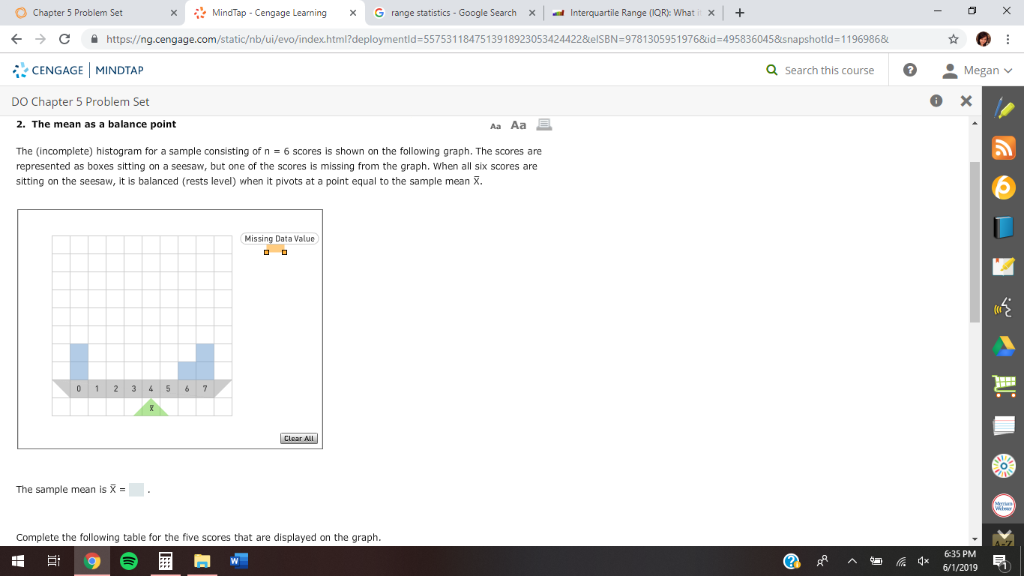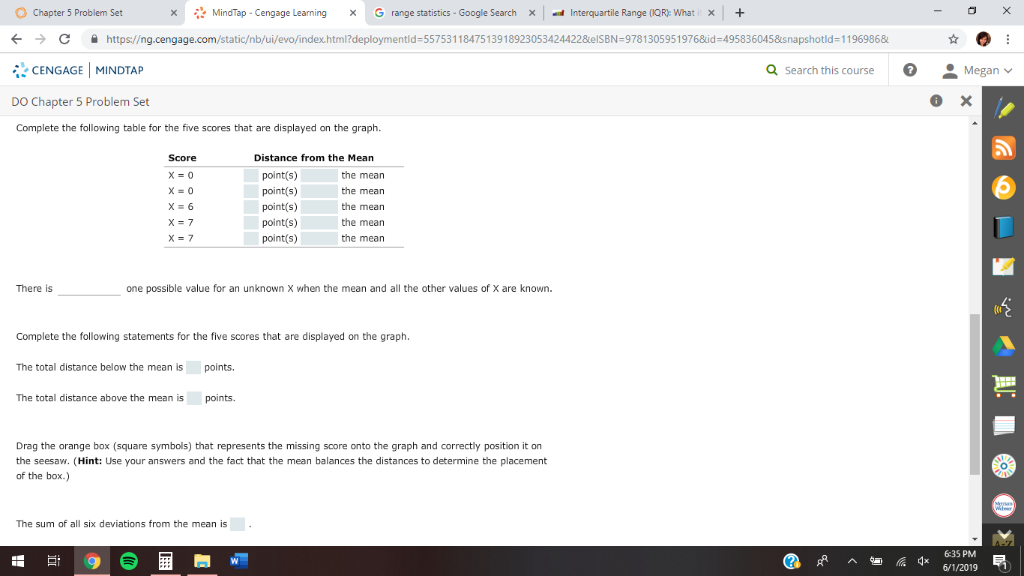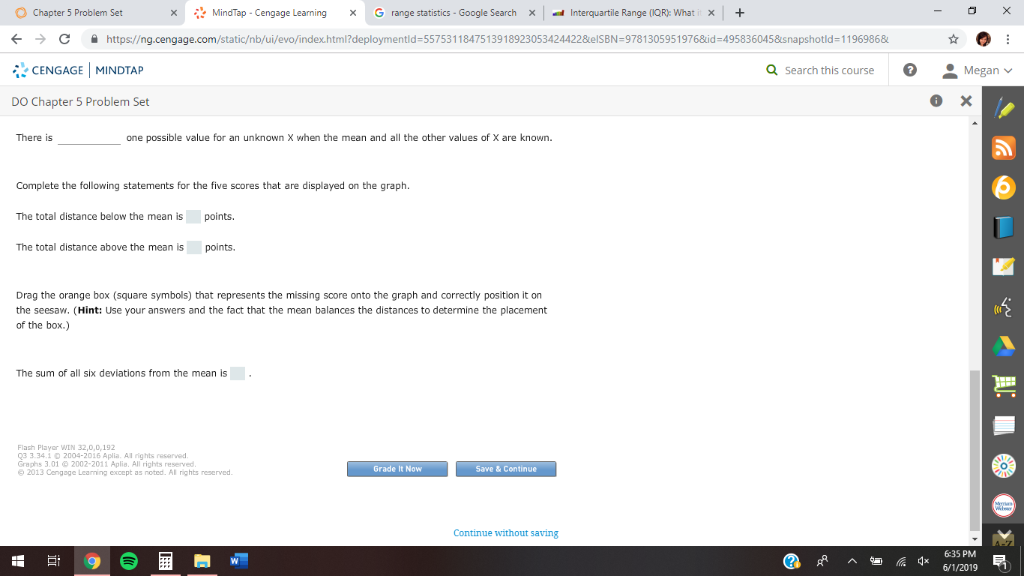MindTap Cengage Learning Interquartile Range (IQR): What it x G range statistics - Google Search x Chapter 5 Problem Set X https://ng.cengage.com/static/nb/ui/evo/index.html1?deploymentId=55753118475139189230534244228e IS BN=9781305951976&id =4958360458&s napshotld = 1 196986& CENGAGE MINDTAP Q Search this course Megan X DO Chapter 5 Problem Set 2. The mean as a balance point Aa Aa The (incomplete) histogram for a sample consisting of n represented as boxes sitting on a seesaw, but one of the scores is missing from the graph. When all six scores are sitting on the seesaw, it is balanced (rests level) when it pivots at a point equal to the sample mean . = 6 scores is shown on the following graph. The scores are Missing Data Value 0 12 3 4 56 7 Clear All O. The sample mean is X . Complete the following table for the five scores that are dissplayed on the graph. 6:35 PM 6/1/2019
MindTap Cengage Learning Interquartile Range (IQR): What it x Chapter 5 Problem Set G range statistics - Google Search x X https://ng.cengage.com/static/nb/ui/evo/index.html1?deploymentId=55753118475139189230534244228e IS BN=9781305951976&id =4958360458&s napshotld = 1 196986& CENGAGE MINDTAP Q Search this course Megan X DO Chapter 5 Problem Set Complete the following table for the five scores that are displayed on the graph. Distance from the Mean Score X 0 point(s) the mean x 0 point(s) the mean X = 6 the mean point(s) point(s) the mean X 7 X 7 point(s) the mean There is one possible value for an unknown X when the mean and all the other values of X are known. Complete the following statements for the five scores that are displayed on the graph. points. The total distance below the mean is The total distance above the mean is points Drag the orange box (square symbols) that represents the missing score onto the graph and correctly position it on the seesaw. (Hint: Use your answers and the fact that the mean balances the distances to determine the placement of the hox. The sum of all six deviations from the mean is - 6:35 PM 6/1/2019
MindTap Cengage Learning Interquartile Range (IQR): What it x Chapter 5 Problem Set G range statistics - Google Search x X https://ng.cengage.com/static/nb/ui/evo/index.html1?deploymentId=55753118475139189230534244228e IS BN=9781305951976&id =4958360458&s napshotld = 1 196986& CENGAGE MINDTAP Q Search this course Megan X DO Chapter 5 Problem Set There is one possible value for an unknown when the mean and all the other values of X are known. Complete the following statements for the five scores that are displayed on the graph points. The total distance below the mean is The total distance above the mean is points. Drag the orange box (square symbols) that represents the missing score onto the graph and correctly position it on the seesaw. (Hint: Use your answers and the fact that the mean balances the distances to determine the placement the box.) The sum of all six deviations from the mean is Flach Player WIN 32.0.0.192 Q3 3.34.1 0 2004-2016 Aplila. Al nghis resarved 2013 Ce Rt oted lri Grade It Now Save& Continue eapnd Continue without saving 6:35 PM 6/1/2019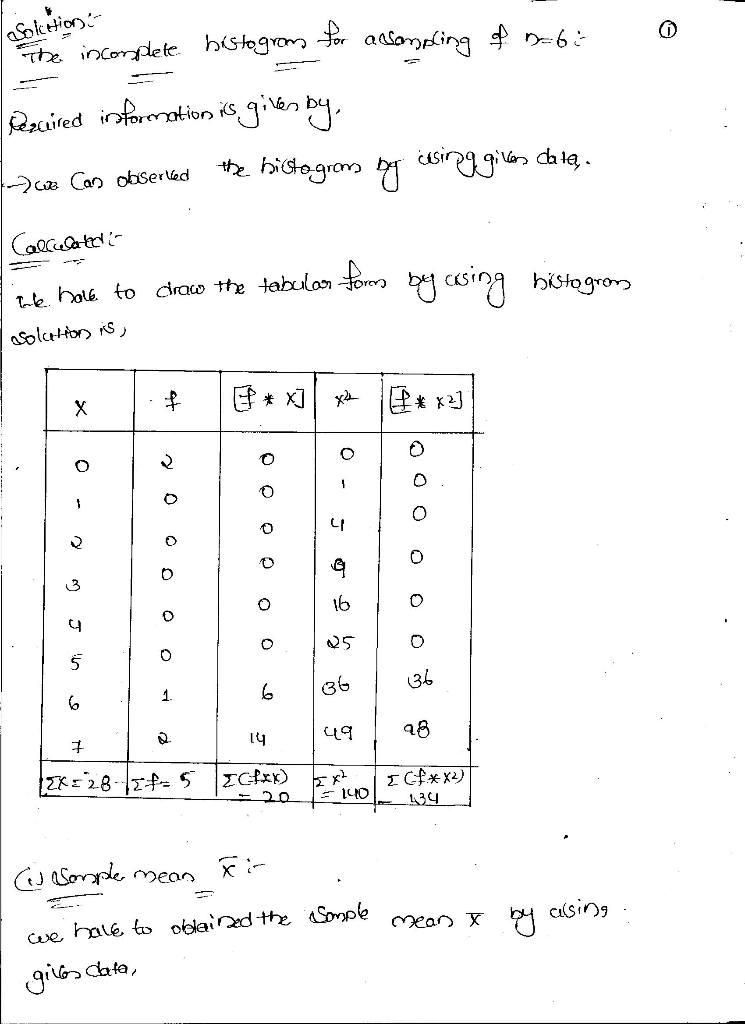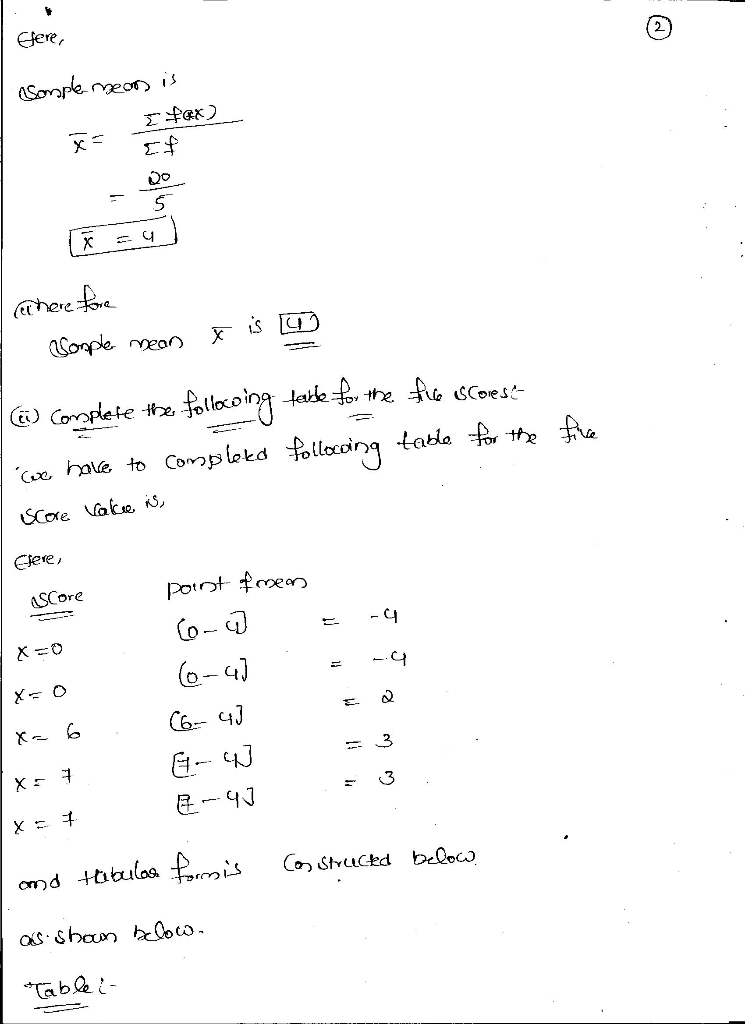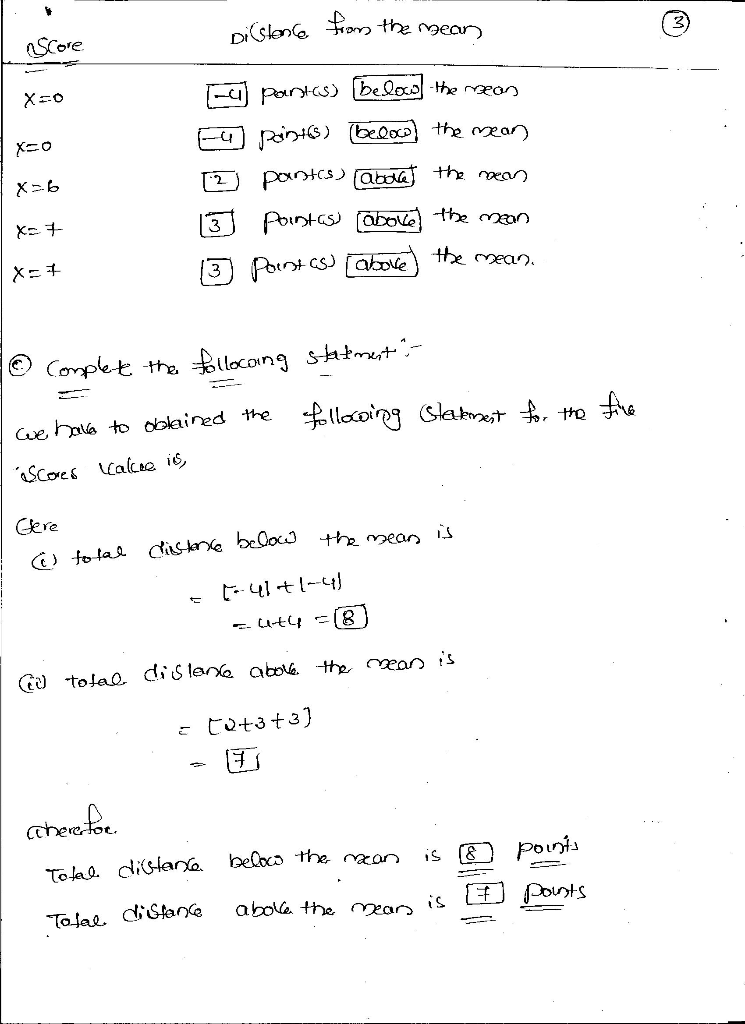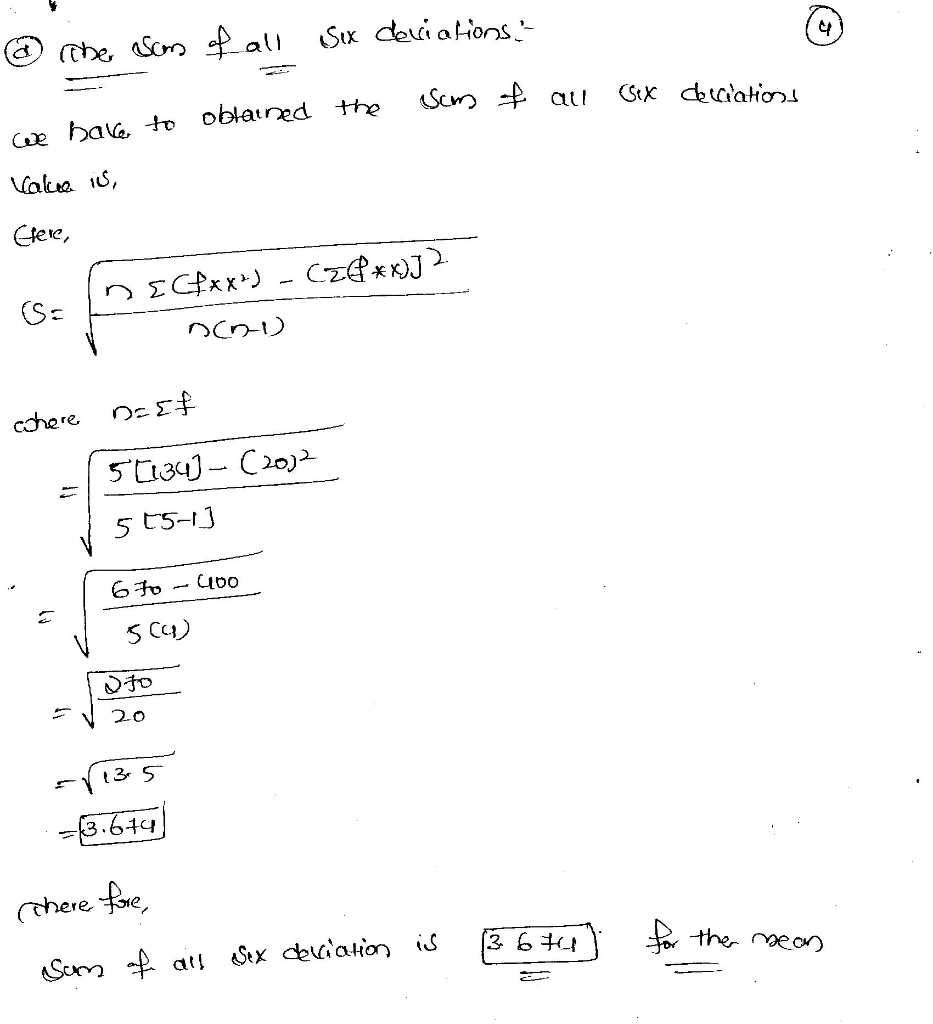##### Add Answer of: MindTap Cengage Learning Interquartile Range (IQR): What it x G range statistics - Google Search x Chapter 5 Problem Se...
More Homework Help Questions Additional questions in this topic.